# DeVos, Matt

## Friendly partitions ★★

Author(s): DeVos

A friendly partition of a graph is a partition of the vertices into two sets so that every vertex has at least as many neighbours in its own class as in the other.

Problem   Is it true that for every, all but finitely many-regular graphs have friendly partitions?

Keywords: edge-cut; partition; regular

## Circular colouring the orthogonality graph ★★

Author(s): DeVos; Ghebleh; Goddyn; Mohar; Naserasr

Letdenote the graph with vertex set consisting of all lines through the origin in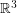and two vertices adjacent inif they are perpendicular.

Problem   Is?

## 5-local-tensions ★★

Author(s): DeVos

Conjecture   There exists a fixed constant(probably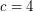suffices) so that every embedded (loopless) graph with edge-width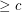has a 5-local-tension.

Keywords: coloring; surface; tension

## Gao's theorem for nonabelian groups ★★

Author(s): DeVos

For every finite multiplicative group, let(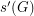) denote the smallest integerso that every sequence ofelements ofhas a subsequence of length(length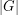) which has product equal to 1 in some order.

Conjecturefor every finite group.

Keywords: subsequence sum; zero sum

## Antichains in the cycle continuous order ★★

Author(s): DeVos

If,are graphs, a functionis called cycle-continuous if the pre-image of every element of the (binary) cycle space ofis a member of the cycle space of.

Problem   Does there exist an infinite set of graphs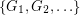so that there is no cycle continuous mapping between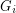andwhenever?

Keywords: antichain; cycle; poset

## Drawing disconnected graphs on surfaces ★★

Author(s): DeVos; Mohar; Samal

Conjecture   Letbe the disjoint union of the graphs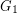andand let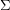be a surface. Is it true that every optimal drawing ofonhas the property thatandare disjoint?

Keywords: crossing number; surface

## What is the largest graph of positive curvature? ★

Author(s): DeVos; Mohar

Problem   What is the largest connected planar graph of minimum degree 3 which has everywhere positive combinatorial curvature, but is not a prism or antiprism?

Keywords: curvature; planar graph

## Partitioning edge-connectivity ★★

Author(s): DeVos

Question   Letbe an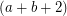-edge-connected graph. Does there exist a partitionof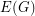so thatis-edge-connected andis-edge-connected?

Keywords: edge-coloring; edge-connectivity

## Packing T-joins ★★

Author(s): DeVos

Conjecture   There exists a fixed constant(probably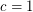suffices) so that every graft with minimum-cut size at leastcontains a-join packing of size at least.

Keywords: packing; T-join

## A homomorphism problem for flows ★★

Author(s): DeVos

Conjecture   Letbe abelian groups and letand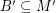satisfy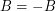and. If there is a homomorphism from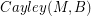to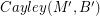, then every graph with a B-flow has a B'-flow.

Keywords: homomorphism; nowhere-zero flow; tension